Next: Plane-Wave Solutions Up: Dirac Equation Previous: Adjoint Spinor

# Bilinear Covariants

The wave functions themselves do not represent observables directly, but one can construct bilinear experessions of the wave functions which have the transformation properties of tensors. All Dirac matrices are simply constants and have the same value in all Lorentz frames. However, when contracted with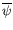and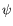different bilinears have their own distinct transformation properties. Some of these have a simple physical interpretation.

The combination of all the gamma matrices occurs often enough that we define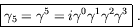(5.164)

We see that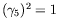,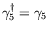, and that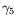anticommutes with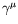: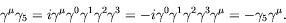(5.165)

Also,commutes with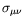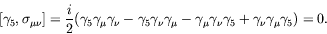(5.166)thus commutes with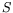for proper Lorentz transformation but anticommutes withfor improper Lorentz transformation. We can write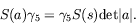(5.167)

By forming products of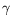matrices it is possible to construct 16 linearly independent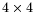matrices,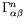: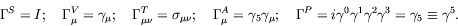(5.168)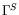has no gamma matrices of which there are one,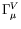has one gamma matric of which there are four,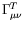has two gamma matrices of which there are six,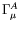has three gamma matrices of which there are four, and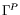has four gamma matrices of which there is one.

We show how the bilinears,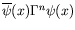, transform under a Lorentz transformation.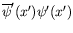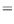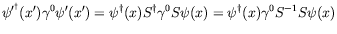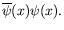(5.169)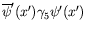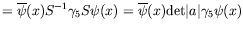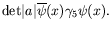(5.170)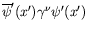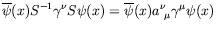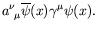(5.171)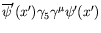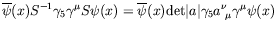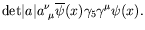(5.172)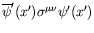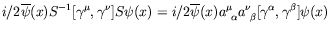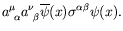(5.173)

Thus the Lorentz transformation properties of these bilinear forms can be summarized as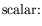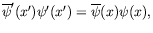(5.174)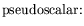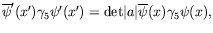(5.175)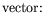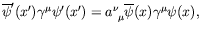(5.176)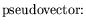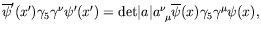(5.177)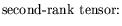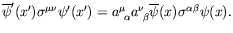(5.178)

Not all of these covariant forms are realized in electromagnetic scattering theory. In particle physics theories of the weak interaction, and the less established theories involving gravity and super-symmetry, a rich set of bilinear forms are used. Which forms are realized in nature is still an ongoing field of research in particle physics.Next: Plane-Wave Solutions Up: Dirac Equation Previous: Adjoint Spinor
Douglas M. Gingrich (gingrich@ ualberta.ca)
2004-03-18